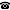# 2011 seminar talk: aleph_1 perfect mad families

Talk held by Jörg Brendle (Kobe University, Japan) at the KGRC seminar on 2011-09-19.

### Abstract

We investigate the complexity of maximal almost disjoint (mad) families of subsets of omega. A classical theorem of Mathias says that there are no analytic mad families. On the other hand, Miller proved that there are coanalytic mad families in the constructible universe $$L$$. By forcing with a p.o. preserving such a family over $$L$$, one sees that the existence of coanalytic mad families is consistent with non-CH. Friedman and Zdomskyy proved that the existence of a $$\Pi^1_2$$ mad family is consistent with $$b > \aleph_1$$, and asked whether the complexity could be improved to $$\Sigma^1_2$$ in their result. In joint work with Yurii Khomskii, we prove that this is indeed the case. (We even conjecture that coanalytic mad families are consistent with $$b > \aleph_1$$, though we still do not have a proof for that.) More explicitly, we show that, under CH, one can construct a sequence of $$\aleph_1$$ many perfect almost disjoint sets whose union is almost disjoint as well and which survives after adding dominating reals. Under $$V = L$$, this sequence, as well as the set defined from it, has a $$\Sigma^1_2$$ definition.

Kurt Gödel Research Center for Mathematical Logic. Währinger Straße 25, 1090 Wien, Austria.+43-1-4277-50501. Last updated: 2010-12-16, 04:37.